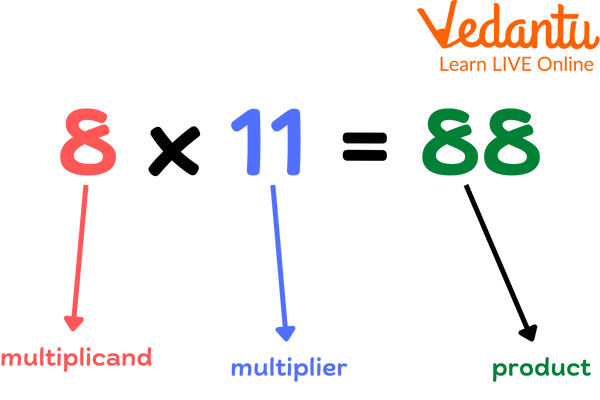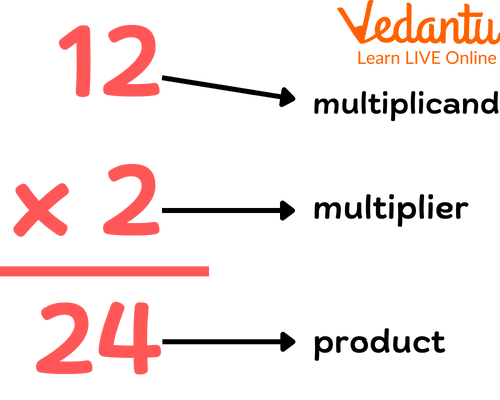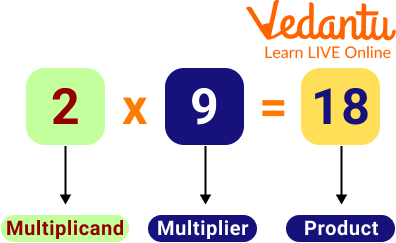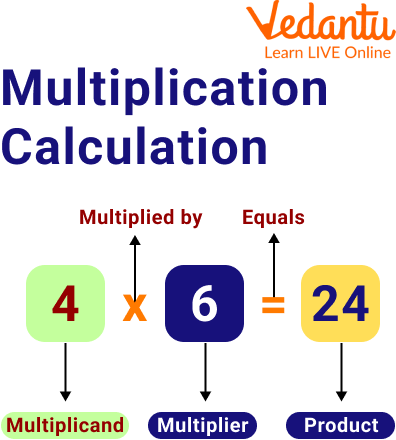Courses
Courses for Kids
Free study material
Free LIVE classes
More

# Multiplicand and MultiplierLIVE
Join Vedantu’s FREE Mastercalss

## What are Multiplicands and Multipliers?

Mathematical expressions are pretty awesome. They are super logical and organised when you look into them. Every expression (Any sequence of numbers with a bunch of symbols can be called a mathematical expression) has an order, each part has a particular name.Parts of Multiplication

When it comes to multiplication, every number used has a name. If you have guessed by now, parts of a multiplication consist of multiplicand, multiplier and product. We will learn more about these new terms Multiplicand, Multipliers and products with various multiplier examples in this article.

## What is Multiplicand in Maths?

The number to be multiplied with the given number is called a multiplicand. Though it is a bit confusing, remembering multiplicand to be the number before the multiplication symbol makes it a bit easier. Let's take the example of Multiplicand below.

In the equation 4 x 5 = 20, 4 is the multiplicand or we can also say that 4 is the mathematical expression of the Multiplicand.Multiplicand

## What is Multiplier in Maths?

The number which is multiplied by the given multiplicand is called a multiplier. It is easier to remember the multiplier to be the number after the multiplication symbol to ease confusion while marking the parts of multiplication. Let's take an example of a Multiplier below.

In the equation 4 x 5 = 20, 5 is the multiplier, we can also say that 5 is the mathematical expression of the Multiplier.

A multiplier is a number that is multiplied to increase the value of the multiplicand at the end of solving an equation.Multiplier, Multiplicand and Product

Above mentioned image shows the mathematical expression of the multiplier.

## What is a Product?

The answer obtained at the end of a multiplication equation is called the product. The result obtained when a multiplicand and a multiplier are multiplied is called the product. It is important to remember that product is nothing but the increased multiplicand obtained at the end of the process of multiplication.

In the equation 4 x 5 = 20, 20 is the product.Product

## Difference between Multiplier and Multiplicand

The "factors" are often the numbers that need to be multiplied. The "multiplicand" is the number that has to be multiplied, and the "multiplier" is the number by which it is multiplied.

For example, each of the three groupings in this instance has six candies. There are therefore 3 times 6 candies, or 6 + 6 + 6, for a total of 18. The result of multiplying two numbers is the "product." The term "multiplicand" refers to the number of items in each group whereas the term "multiplier" refers to the quantity of such equal groups.

## Solved Examples

1. Find the product for an expression where the multiplicand is 40 and the multiplier is 3.

Ans: The given statement can be re-written as 40 x 3 where 40 is the multiplicand and 3 is the multiplier.

To solve the expression, 40 x 3= 120.

So, the product of the given expression is 120.

2. For a given expression “50 x 2= 100”, find the parts of the multiplication.

Ans: 50 is the multiplicand.

2 is the multiplier.

100 is the product of the expression.

3. Find the product for an expression where the multiplicand is 127 and the multiplier is 7.

Ans: The given statement can be re-written as 127 x 7 where 127 is the multiplicand and 7 is the multiplier.

To solve the expression, 127 x 7= 889

So, the product of the given expression is 889.

4. For a given expression “193 x 2= 386”, find the parts of the multiplication.

Ans: 193 is the multiplicand.

2 is the multiplier.

386 is the product of the expression.

5. Find the product for an expression where the multiplicand is 39 and the multiplier is 9.

Ans: The given statement can be re-written as 39 x 9 where 39 is the multiplicand and 9 is the multiplier.

So, the product of the given expression is 39 x 9 = 351.

## Practice Problems

1. Multiply 34 by 4 and identify the parts of the multiplication.

Ans: 34 x 4 = 136. 34 is the multiplicand, 4 is the multiplier, and 136 is the product.

2. Find the product for an expression where the multiplicand is 80 and the multiplier is 6.

Ans: The product of the expression is 480.

3. For a given expression “74 x 8= 592”, find the parts of the multiplication.

Ans: 74 is the multiplicand, 8 is the multiplier, and 592 is the product.

4. Multiply 89 by 6 and identify the parts of the multiplication.

Ans: 89 is the multiplicand, 6 is the multiplier and 534 is the product.

5. Find the product for an expression where the multiplicand is 72 and the multiplier is 5.

Ans: The product of the expression is 360.

## Summary

Let’s brush through what we’ve learnt up until now. Multiplication has a number of parts like the multiplicand, multiplier and product. The multiplicand is the number to which another number is multiplied and whose value is observed to increase. A multiplier is a number which is multiplied by the multiplicand. The result obtained by the multiplication of multiplicand and multiplier is called the product of the multiplication. These parts are important to be identified and understood in order to ensure better application of multiplication expressions in Mathematics.

Last updated date: 25th Sep 2023
Total views: 94.2k
Views today: 1.94k

## FAQs on Multiplicand and Multiplier

1. What are the factors and multiples?

Factors, multiples and multiplication are closely related. A factor is defined as a number that divides the given number without leaving a remainder. For example, 3 is a factor of 9. A multiple is the number obtained when multiplying 2 factors.

2. How do positive and negative signs of the multiplier and multiplicand affect the product?

This is a pretty high-level idea to deal with the given scope of this article. But yes, dealing with signs in multiplication can be a bit tricky. For easier understanding, we’ll assume that the multiplicand is always positive. If the multiplier is negative, the product obtained is going to be negative and if the multiplier is positive, then the product obtained is going to be positive. In conclusion, if one of the multiplier and multiplicand is of negative signs, then the value of the product is negative.

3. Define factors and multiple in relation to parts of multiplication.

In relation to parts of multiplication, multiplicand and multiplier are factors in the given expression. Product is multiple in the obtained expression. These terms are related to multiplication slightly, but understanding the basic concept of factors and multiples proves sufficient.